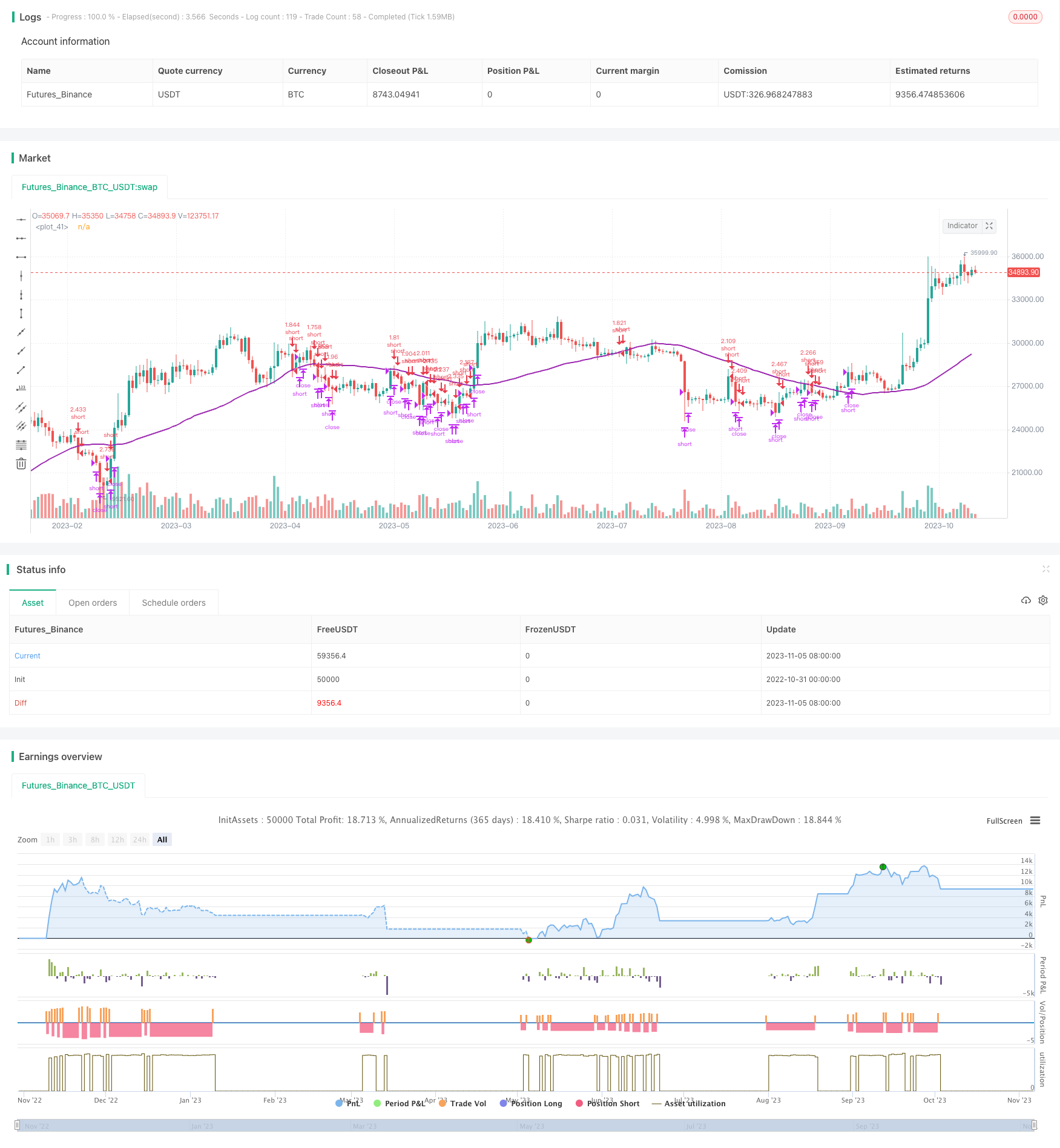# Short Trading Strategy in Downtrend

Author: ChaoZhang, Date: 2023-11-07 17:06:59
Tags:## Overview

This strategy takes advantage of downtrend by building short positions gradually based on moving average and RSI indicators. It aims for profit in falling market.

## Strategy Logic

When close price is below 100-day simple moving average and RSI is greater than 30, go short. Then set stop loss above entry price by 3% and take profit below entry price by 2%. The wider stop loss allows more volatility to avoid unnecessary stops. Close position when price surpasses stop loss or falls below take profit.

On Coinrule platform, set multiple sequential sell orders to build position gradually. When downtrend persists, increase position size. Setting a time interval between orders also helps controlling overall position size.

Each trade is connected with a stop loss order and take profit order. The percentages are optimized for mid-cap coins. You can adjust based on specific coin. As it trades along with trend, stop loss and take profit ratio like 1:1.5 could work.

Stop loss at 3% above entry price Take profit at 2% below entry price A slightly larger stop loss tolerates more volatility.

• MA judges trend direction well, catching downtrend in time
• RSI filter avoids blindly going short
• Gradual position build controls risk maximally with better risk-reward ratio
• Stop loss and take profit ensure endurance for each trade

## Risk Analysis

• Sharp V reversal could lead to major loss
• Need to monitor price closely to adjust stop loss and take profit
• Leverage needs to be reasonable to control position size
• Pausing strategy in choppy market avoids unnecessary loss

## Optimization Directions

• Test MA with different parameters
• Test RSI combinations with different parameters
• Adjust stop loss and take profit ratios to optimize risk-reward
• Test different time intervals between orders to control position size

## Summary

This strategy effectively captures downtrend based on MA and RSI. Gradual position build controls risk while stop loss and take profit ensure endurance. Further optimizing risk-reward ratio by adjusting stop loss/take profit parameters. There is room for improvements on parameters and risk control. But overall a solid short-term short strategy.

```/*backtest
start: 2022-10-31 00:00:00
end: 2023-11-06 00:00:00
period: 1d
basePeriod: 1h
exchanges: [{"eid":"Futures_Binance","currency":"BTC_USDT"}]
*/

// This source code is subject to the terms of the Mozilla Public License 2.0 at https://mozilla.org/MPL/2.0/

//@version=4
strategy(shorttitle='Short In Downtrend',title='Short In Downtrend Below MA100', overlay=true, initial_capital = 1000, process_orders_on_close=true, default_qty_type = strategy.percent_of_equity, default_qty_value = 100)

//Backtest dates
fromMonth = input(defval = 1,    title = "From Month",      type = input.integer, minval = 1, maxval = 12)
fromDay   = input(defval = 10,    title = "From Day",        type = input.integer, minval = 1, maxval = 31)
fromYear  = input(defval = 2019, title = "From Year",       type = input.integer, minval = 1970)
thruMonth = input(defval = 1,    title = "Thru Month",      type = input.integer, minval = 1, maxval = 12)
thruDay   = input(defval = 1,    title = "Thru Day",        type = input.integer, minval = 1, maxval = 31)
thruYear  = input(defval = 2112, title = "Thru Year",       type = input.integer, minval = 1970)

showDate  = input(defval = true, title = "Show Date Range", type = input.bool)

start     = timestamp(fromYear, fromMonth, fromDay, 00, 00)        // backtest start window
finish    = timestamp(thruYear, thruMonth, thruDay, 23, 59)        // backtest finish window
window()  => true       // create function "within window of time"

//MA inputs and calculations
inSignal=input(50, title='MASignal')

MA= sma(close, inSignal)

// RSI inputs and calculations
lengthRSI = input(14, title = 'RSI period', minval=1)
RSI = rsi(close, lengthRSI)

//Entry
strategy.entry(id="short", long = false, when = close < MA and RSI > 30)

//Exit
shortStopPrice  = strategy.position_avg_price * (1 + 0.03)
shortTakeProfit = strategy.position_avg_price * (1 - 0.02)

strategy.close("short", when = close > shortStopPrice or close < shortTakeProfit and window())

plot(MA, color=color.purple, linewidth=2)

```

More